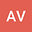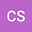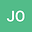Interacting wind- and tide-forced boundary-layers in a large strait
•••• +1
Arnaud François ValcarcelUniversity of Otago / National Institute for Water & Atmospheric Research, University of Otago / National Institute for Water & Atmospheric Research

Corresponding Author:arnaud.valcarcel@niwa.co.nz

Author ProfileCraig L. StevensNational Institute for Water & Atmospheric Research, Wellington, New Zealand, National Institute for Water & Atmospheric Research, Wellington, New Zealand
Author ProfileJoanne O'CallaghanNational Institute of Water and Atmospheric Research, National Institute of Water and Atmospheric Research
Author Profile## Abstract

Observations of the spatio-temporal structure of turbulent mixing in a large, energetic strait were used to examine the interactions between wind- and tidally-forced boundary layers in a coastal environment. Te Moana-o-Raukawa (Cook Strait) of Aotearoa (New Zealand) is a relatively wide, energetic strait, known to experience substantial tidal currents and wind stress. A turbulence-enabled ocean glider mission measured $\mathcal{O}(40,000)$ turbulence samples that passed QAQC including the use of a vehicle-mounted speed through water sensor. The observations were compared to one-dimensional models of turbulence to understand the mechanisms that regulates the vertical structure of mixing. Tidal flows of $\mathcal{O}(\SI{1}{\meter\per\second})$ and wind speeds of $\mathcal{O}(\SI{10}{\meter\per\second})$ enhance dissipation to $\epsilon=\mathcal{O}(10^{-5}\SI{}{\watt\per\kilogram})$ through boundary drag, shear-driven production of turbulent kinetic energy ($P$) and to a minor extent buoyancy flux ($G$). The benthic and wind-driven boundary layers behaved reasonably predictably when considering a 1D perspective. The interaction between the two boundary layers depended on mid-water column stratification which is to a large degree an externally-prescribed condition. Transient stratification can stabilize the mean flow (median $Ri_g=0.6(>1/4)$) and reduce both turbulence intensity ($Re_b$) and diapycnal diffusivity ($K_z$) by up to two orders of magnitude in the middle of the water column, insulating bottom and surface mixing-layers. Mid-water dissipation rate levels tend to be associated with marginal dynamical stability (median $Ri_g=0.22(\sim1/4)$) and canonical mixing efficiency (median $R_f=0.17$), while elevated levels are connected to unstable mean flow conditions (median $Ri_g=0.14(<1/4)$) and reduced mixing efficiency (median $R_f=0.1(<0.17)$) that promotes turbulence growth.